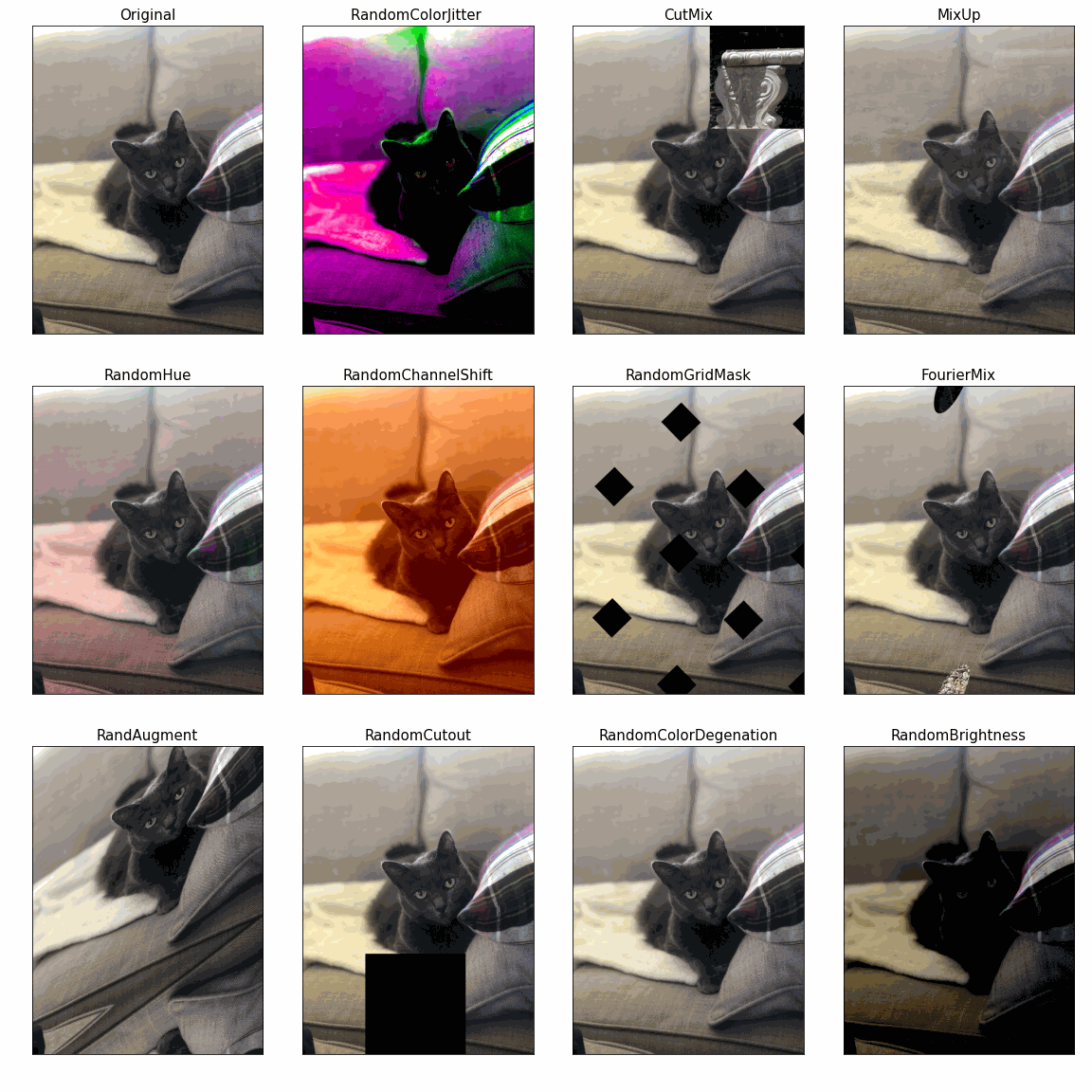» KerasCV

# KerasCV

KerasCV is a toolbox of modular building blocks (layers, metrics, losses, data augmentation) that computer vision engineers can leverage to quickly assemble production-grade, state-of-the-art training and inference pipelines for common use cases such as image classification, object detection, image segmentation, image data augmentation, etc.## Installation

KerasCV requires Python 3.7+ and TensorFlow 2.9+.

Install the latest release:

``````pip install keras-cv --upgrade
``````

You can also check out other versions in our GitHub repository.

## Quick Introduction

Create a preprocessing pipeline:

``````import keras_cv
import tensorflow as tf
from tensorflow import keras
import tensorflow_datasets as tfds

preprocessing_layers = [
keras_cv.layers.RandomResizedCrop(
target_size=(224, 224),
crop_area_factor=(0.8, 1.0),
aspect_ratio_factor=(3/4, 4/3)
),
keras_cv.layers.RandomFlip(),
keras_cv.layers.RandAugment(value_range=(0, 255)),
keras_cv.layers.CutMix(),
keras_cv.layers.MixUp()
]

def augment_data(images, labels):
labels = tf.one_hot(labels, 3)
inputs = {"images": images, "labels": labels}
for layer in preprocessing_layers:
inputs = layer(inputs)
return inputs['images'], inputs['labels']
``````

Augment a `tf.data.Dataset`:

``````dataset = tfds.load('rock_paper_scissors', as_supervised=True, split='train')
dataset = dataset.batch(64)
dataset = dataset.map(augment_data)
``````

Create a model:

``````densenet = keras_cv.models.DenseNet121(
include_rescaling=True,
include_top=True,
classes=3
)
densenet.compile(
loss='categorical_crossentropy',
metrics=['accuracy']
)
``````

``````densenet.fit(dataset)
``````

## Citing KerasCV

If KerasCV helps your research, we appreciate your citations. Here is the BibTeX entry:

``````@misc{wood2022kerascv,
title={KerasCV},
author={Wood, Luke and Zhu, Scott and Chollet, Fran\c{c}ois and others},
year={2022},
howpublished={\url{https://github.com/keras-team/keras-cv}},
}
``````